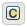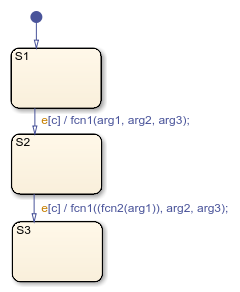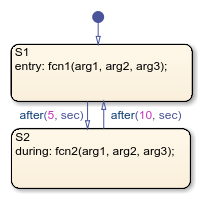Call C Library Functions in C Charts

Stateflow® charts in Simulink® models have an action language property that defines the syntax for state and transition actions. An icon in the lower-left corner of the chart canvas indicates the action language for the chart.

•MATLAB® as the action language.

•C as the action language.

Call C Library Functions

You can call this subset of the C Math Library functions:

 abs* ** acos** asin** atan** atan2** ceil** cos** cosh** exp** fabs floor** fmod** labs ldexp** log** log10** pow** rand sin** sinh** sqrt** tan** tanh** * The Stateflow abs function goes beyond that of its standard C counterpart with its own built-in functionality. For more information, see Call the abs Function. ** You can also replace calls to the C Math Library with application-specific implementations for this subset of functions. For more information, see Replacement of Math Library Functions with Application Implementations.

When you call these functions, double precision applies unless all the input arguments are explicitly single precision. When a type mismatch occurs, a cast of the input arguments to the expected type replace the original arguments. For example, if you call the sin function with an integer argument, a cast of the input argument to a floating-point number of type double replaces the original argument.

Note

Because the input arguments to the C library functions are first cast to floating-point numbers, function calls with arguments of type int64 or uint64 can result in loss of precision.

If you call other C library functions not listed above, include the appropriate #include... statement in the Simulation Target pane of the Configuration Parameters.

Call the abs Function

Interpretation of the Stateflow abs function goes beyond the standard C version to include integer and floating-point arguments of all types as follows:

• If x is an integer of type int32 or int64, the standard C function abs applies to x, or abs(x).

• If x is an integer of type int16 or int8, the standard C abs function applies to a cast of x as an integer of type int32, or abs((int32)x).

• If x is a floating-point number of type double, the standard C function fabs applies to x, or fabs(x).

• If x is a floating-point number of type single, the standard C function fabs applies to a cast of x as a double, or fabs((double)x).

• If x is a fixed-point number, the standard C function fabs applies to a cast of the fixed-point number as a double, or fabs((double) Vx), where Vx is the real-world value of x.

If you want to use the abs function in the strict sense of standard C, cast its argument or return values to integer types. See Type Cast Operations.

Note

If you declare x in custom code, the standard C abs function applies in all cases. For instructions on inserting custom code into charts, see Reuse Custom Code in Stateflow Charts.

Call min and max Functions

You can call min and max by emitting the following macros automatically at the top of generated code.

#define min(x1,x2) ((x1) > (x2) ? (x2):(x1))
#define max(x1,x2) ((x1) > (x2) ? (x1):(x2))

To allow compatibility with user graphical functions named min() or max(), generated code uses a mangled name of the following form: <prefix>_min. However, if you export min() or max() graphical functions to other charts in your model, the name of these functions can no longer be emitted with mangled names in generated code and conflict occurs. To avoid this conflict, rename the min() and max() graphical functions.

Replacement of Math Library Functions with Application Implementations

You can configure the code generator to change the code that it generates for math library functions such that the code meets application requirements. To do this you configure the code generator to apply a code replacement library (CRL) during code generation. If you have an Embedded Coder® license, you can develop and apply custom code replacement libraries.

For more information about replacing code, using code replacement libraries that MathWorks® provides, see What Is Code Replacement? (Simulink Coder) and Code Replacement Libraries (Simulink Coder). For information about developing custom code replacement libraries, see What Is Code Replacement Customization? (Embedded Coder) and Code You Can Replace From Simulink Models (Embedded Coder).

Call Custom C Code Functions

You can specify custom code functions for use in C charts for simulation and C code generation.

Specify Custom C Functions for Simulation

To specify custom C functions for simulation:

1. Open the Model Configuration Parameters dialog box.

2. Select Simulation Target.

3. Specify your custom C files, as described in Reuse Custom Code in Stateflow Charts.

Specify Custom C Functions for Code Generation

To specify custom C functions for code generation:

1. Open the Model Configuration Parameters dialog box.

2. Select Code Generation > Custom Code.

3. Specify your custom C files, as described in Integrate External Code for All Charts (Simulink Coder).

Guidelines for Calling Custom C Functions in Your Chart

• Define a function by its name, any arguments in parentheses, and an optional semicolon.

• Pass parameters to user-written functions using single quotation marks. For example, func('string').

• An action can nest function calls.

• An action can invoke functions that return a scalar value (of type double in the case of MATLAB functions and of any type in the case of C user-written functions).

Guidelines for Writing Custom C Functions That Access Input Vectors

• Use the sizeof function to determine the length of an input vector.

For example, your custom function can include a for-loop that uses sizeof as follows:

for(i=0; i < sizeof(input); i++) {
......
}
• If your custom function uses the value of the input vector length multiple times, include an input to your function that specifies the input vector length.

For example, you can use input_length as the second input to a sum function as follows:

int sum(double *input, double input_length)

Your sum function can include a for-loop that iterates over all elements of the input vector:

for(i=0; i < input_length; i++) {
......
}

Function Call in Transition Action

Example formats of function calls using transition action notation appear in the following chart.A function call to fcn1 occurs with arg1, arg2, and arg3 if the following are true:

• S1 is active.

• Event e occurs.

• Condition c is true.

• The transition destination S2 is valid.

The transition action in the transition from S2 to S3 shows a function call nested within another function call.

Function Call in State Action

Example formats of function calls using state action notation appear in the following chart.Chart execution occurs as follows:

1. When the default transition into S1 occurs, S1 becomes active.

2. The entry action, a function call to fcn1 with the specified arguments, executes.

3. After 5 seconds of simulation time, S1 becomes inactive and S2 becomes active.

4. The during action, a function call to fcn2 with the specified arguments, executes.

5. After 10 seconds of simulation time, S2 becomes inactive and S1 becomes active again.

6. Steps 2 through 5 repeat until the simulation ends.

Pass Arguments by Reference

A Stateflow action can pass arguments to a user-written function by reference rather than by value. In particular, an action can pass a pointer to a value rather than the value itself. For example, an action could contain the following call:

f(&x);

where f is a custom-code C function that expects a pointer to x as an argument.

If x is the name of a data item defined in the Stateflow hierarchy, the following rules apply:

• Do not use pointers to pass data items input from a Simulink model.

If you need to pass an input item by reference, for example, an array, assign the item to a local data item and pass the local item by reference.

• If x is a Simulink output data item having a data type other than double, the chart property Use Strong Data Typing with Simulink I/O must be on (see Specify Properties for Stateflow Charts).

• If the data type of x is boolean, you must turn off the coder option Use bitsets for storing state configuration.

• If x is an array with its first index property set to 0 (see Set Data Properties), then you must call the function as follows.

f(&(x));

This passes a pointer to the first element of x to the function.

• If x is an array with its first index property set to a nonzero number (for example, 1), the function must be called in the following way:

f(&(x));

This passes a pointer to the first element of x to the function.

Stateflow DocumentationGet trial now